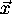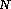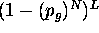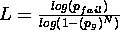# The RANSAC (Random Sample Consensus) Algorithm

Robert B. Fisher

The RANSAC algorithm  is an algorithm for robust fitting of models in the presence of many data outliers. The algorithm is very simple.

Given a fitting problem with parameters, estimate the parameters.

Assume:

1. The parameters can be estimated from N data items.
2. There are M data items in total.
3. The probability of a randomly selected data item being part of a good model is.
4. The probability that the algorithm will exit without finding a good fit if one exists is.

Then, the algorithm:

1. selects N data items at random
2. estimates parameter3. finds how many data items (of M) fit the model with parameter vectorwithin a user given tolerance. Call this K.
4. if K is big enough, accept fit and exit with success.
5. repeat 1..4 L times
6. fail if you get here

How big K has to be depends on what percentage of the data you think belongs to the structure being fit and how many structures you have in the image. If there are multiple structures then, after a successful fit, remove the fit data and redo RANSAC.

You can find L by:= probability of L consecutive failures= (prob that a given trial is a failure)= (1 - prob that a given trial is a success)= (1 - (prob that a random data item fits the model))=Solve for.

1
M. A. Fischler, R. C. Bolles. Random Sample Consensus: A Paradigm for Model Fitting with Applications to Image Analysis and Automated Cartography. Comm. of the ACM, Vol 24, pp 381-395, 1981.

Bob Fisher
Mon May 6 18:05:39 BST 2002